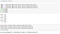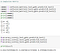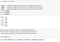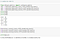# Divorce Prediction Using Machine Learning

In this blog, I will go through the whole process of creating a machine learning model on the divorce predictors dataset. It provides information on the divorce prediction.

# The Dataset

The Dataset is from UCIMachinelearning and it provides you all the relevant information needed for the prediction of Divorce. It contains 54 features and on the basis of these features we have to predict that the couple has been divorced or not. Value 1 represent Divorced and value 0 represent not divorced. Features are as follows:-

1. When one of our apologies apologizes when our discussions go in a bad direction, the issue does not extend.

The dataset itself contains 170 rows and 55 columns.

# Data Preprocessing

We converted our dataset into pandas data frame. Then we created 2 different dataset from this dataset one is the data values and another is the label of the data values names as x and y. Using slicing method in python. Then we divided our dataset into training and testing dataset. In the ratio of 80–20,70–30,60–40.

# Building Machine Learning Models

Random Forest:

Firstly we took random forest algorithm from scikit learn. Then we fit the data in three different ratio 80–20, 70–30, 60–40.

Confusion Matrix & Accuracy for this modelAccuracy In confusion matrix using random forest classifier on 3 different splits

Logistic Regression:

We took Logistic Regression algorithm from scikit learn. Then we fit the data in three different ratio 80–20, 70–30, 60–40.

Confusion Matrix & Accuracy for this modelAccuracy In confusion matrix using logistic regression classifier on 3 different splits

Neural Networks:

For neural network we used the keras library.

Then we fit the data in 80–20 and then we build our model

Naïve Bayes:

We took Naive Bayes algorithm from scikit learn. Then we fit the data in three different ratio 80–20, 70–30, 60–40.

Confusion Matrix & Accuracy for this ModelAccuracy In confusion matrix using Naive Bayes classifier on 3 different splits

Support Vector Machine:

We took SVM algorithm from scikit learn. Then we fit the data in three different ratio 80–20, 70–30, 60–40.

Confusion Matrix & Accuracy for this modelAccuracy In confusion matrix using SVM classifier on 3 different splits

CART Decision Tree:

We took Decision Tree algorithm from scikit learn. Then we fit the data in three different ratio 80–20, 70–30, 60–40.

Confusion Matrix & Accuracy for this modelAccuracy In confusion matrix using random Decision tree on 3 different splits

Perceptron:

We took Perceptron algorithm from scikit learn. Then we fit the data in three different ratio 80–20, 70–30, 60–40.

Confusion Matrix & Accuracy:

# Evaluation Metrics

1. Accuracy - Accuracy is the ratio of number of correct predictions to the total number of input samples. So accuracy for each model has been displayed for each.

Like i have taken the confusion matrix of Random Forest Classifier.

The first row is about the not-divorced-predictions: 19 couples were correctly classified as not divorced (called true negatives) and 0 were wrongly classified as not divorced (false positives).The second row is about the divorced-predictions: 2 couples were wrongly classified as divorced (false negatives) and 13 were correctly classified as divorced (true positives).

So we have successfully trained our dataset into different models for predicting whether a couple will get divorced or not. And also got the accuracy & confusion matrix for each model as well.

I’m providing link for the code so if you want to check then check it out:- https://drive.google.com/drive/folders/12cu1sXZvCQk57qu5TyMYZ7YgRTJ26GM8?usp=sharing